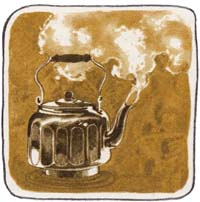Introduction8
###### Sample Problems
The HP-41C display contains seven ‘‘annunciators’’ or key words that tell you the status of the calculator.

 BAT USER GRAD SHIFT 01234 PRGM ALPHA

Press the ON key now and check to see if the USER annunciator in the display is on. If the USER annunciator is displayed, press the USER key (located just below the display) to turn the USER annunciator off.
If the BAT (battery) annunciator is displayed, or your HP-41C does not have the batteries installed, refer to Batteries, page 240.
To get the feel of your new calculator try a few simple calculations. Press g FIX 4 now so your display will match the displays in the following problems.
 To solve: Keystrokes Display 5 + 6 = 11 5 ENTER  6  + 11.0000 8 ÷ 2 = 4 8 ENTER  2  ÷ 4.0000 7 – 4 = 3 7 ENTER  4  – 3.0000 9 × 8 = 72 9 ENTER  8  × 72.0000 19.852 19.85 g X2 394.0225
Now let’s look at a sample problem to see how the HP-41C is used to solve the problem manually and then automatically using a program.
Most convential home water heaters are cylindrical in shape, and you can easily calculate the heat loss from such a water heater. The formula q = hAT can be used, where

 q is the heat loss from the water heater (Btu per hour), h is the heat-transfer coefficient, A is the total surface area of the cylinder, and T is the temperature difference between the cylinder surface and the surrounding air.For our example let’s assume you gave a 52-gallon cylindrical water heater and you wish to determine how much energy is being lost because of poor insulation. In initial measurements, you found an average temperature difference between the heater surface and surrounding air of 15 degrees Fahrenheit. The surface area of the tank is 30 square feet and the heat transfer coefficient is approximately 0.47.